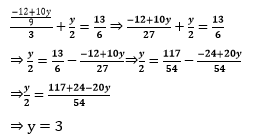Guru

# 1. Solve the following pair of linear equations by the substitution method (i) x + y = 14 x – y = 4 (ii) s – t = 3 (s/3) + (t/2) = 6 (iii) 3x – y = 3 9x – 3y = 9 (iv) 0.2x + 0.3y = 1.3 0.4x + 0.5y = 2.3 (v) √2 x+√3 y = 0 √3 x-√8 y = 0 (vi) (3x/2) – (5y/3) = -2 (x/3) + (y/2) = (13/6) Q.1

• 0

The question of linear equations how can i solve this question by substitution method. I think it is also the most important question of 10Th math of chapter pair of linear equations in two variables the question is 1. Solve the following pair of linear equations by the substitution method (i) x + y = 14 x – y = 4 (ii) s – t = 3 (s/3) + (t/2) = 6 (iii) 3x – y = 3 9x – 3y = 9 (iv) 0.2x + 0.3y = 1.3 0.4x + 0.5y = 2.3 (v) √2 x+√3 y = 0 √3 x-√8 y = 0 (vi) (3x/2) – (5y/3) = -2 (x/3) + (y/2) = (13/6)

Share

1. (i) Given,

x + y = 14 and x – y = 4 are the two equations.

From 1st equation, we get,

x = 14 – y

Now, substitute the value of x in second equation to get,

(14 – y) – y = 4

14 – 2y = 4

2y = 10

Or y = 5

By the value of y, we can now find the exact value of x;

∵ x = 14 – y

∴ x = 14 – 5

Or x = 9

Hence, x = 9 and y = 5.

(ii) Given,

s – t = 3 and (s/3) + (t/2) = 6 are the two equations.

From 1st equation, we get,

s = 3 + t ________________(1)

Now, substitute the value of s in second equation to get,

(3+t)/3 + (t/2) = 6

⇒ (2(3+t) + 3t )/6 = 6

⇒ (6+2t+3t)/6 = 6

⇒ (6+5t) = 36

⇒5t = 30

⇒t = 6

Now, substitute the value of t in equation (1)

s = 3 + 6 = 9

Therefore, s = 9 and t = 6.

(iii) Given,

3x – y = 3 and 9x – 3y = 9 are the two equations.

From 1st equation, we get,

x = (3+y)/3

Now, substitute the value of x in the given second equation to get,

9(3+y)/3 – 3y = 9

⇒9 +3y -3y = 9

⇒ 9 = 9

Therefore, y has infinite values and since, x = (3+y) /3, so x also has infinite values.

(iv) Given,

0.2x + 0.3y = 1.3 and 0.4x + 0.5y = 2.3are the two equations.

From 1st equation, we get,

x = (1.3- 0.3y)/0.2 _________________(1)

Now, substitute the value of x in the given second equation to get,

0.4(1.3-0.3y)/0.2 + 0.5y = 2.3

⇒2(1.3 – 0.3y) + 0.5y = 2.3

⇒ 2.6 – 0.6y + 0.5y = 2.3

⇒ 2.6 – 0.1 y = 2.3

⇒ 0.1 y = 0.3

⇒ y = 3

Now, substitute the value of y in equation (1), we get,

x = (1.3-0.3(3))/0.2 = (1.3-0.9)/0.2 = 0.4/0.2 = 2

Therefore, x = 2 and y = 3.

(v) Given,

√2 x + √3 y = 0 and √3 x – √8 y = 0

are the two equations.

From 1st equation, we get,

x = – (√3/√2)y __________________(1)

Putting the value of x in the given second equation to get,

√3(-√3/√2)y – √8y = 0 ⇒ (-3/√2)y- √8 y = 0

⇒ y = 0

Now, substitute the value of y in equation (1), we get,

x = 0

Therefore, x = 0 and y = 0.

(vi) Given,

(3x/2)-(5y/3) = -2 and (x/3) + (y/2) = 13/6 are the two equations.

From 1st equation, we get,

(3/2)x = -2 + (5y/3)

⇒ x = 2(-6+5y)/9 = (-12+10y)/9 ………………………(1)

Putting the value of x in the given second equation to get,

((-12+10y)/9)/3 + y/2 = 13/6

⇒y/2 = 13/6 –( (-12+10y)/27 ) + y/2 = 13/6Now, substitute the value of y in equation (1), we get,

(3x/2) – 5(3)/3 = -2

⇒ (3x/2) – 5 = -2

⇒ x = 2

Therefore, x = 2 and y = 3.

• 1